# MCQs on Control Systems

##### Page 7 of 23. Go to page 1 2 3 4 5 6 7 8 9 10 11 12 13 14 15 16 17 18 19 20 21 22 23
01․ The open loop transfer function for unity feedback system is given by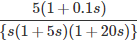Find the steady state error for a ramp input of magnitude 10?
0
2
5
infinite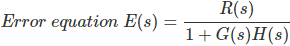Applying final value theorem, Steady state error = Lts→0 S E(s) Steady state error for different types and different inputs as follows: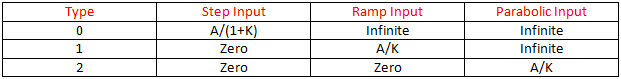02․ The system function N(s) = V(s)/I(s) = (s+3)/(4s+5). The system is initially at rest. If the excitation i(t) is a unit step, which of the following is the final value?
1/4
3/5
1
0

Steady state value can be obtained by applying final value theorem to the function. Lims→0 s C(s) = Lims→0 s R(s)H(s) Where, C(s) = Steady state value R(s) = Input H(s) = Network response Final value = Lims→0 s * (s+3)/(4s+5)*1/s = 3/5

03․ Find the Laplace transform for sinωt?
1/s
1/s²
s/(s²+ω²)
ω/(s²+ω²)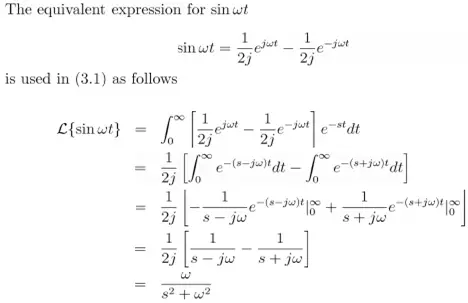04․ Given a unity feedback system with G(s) = k/s(s+4), the value of k for damping ratio of 0.5 is
1
4
16
64

Characteristic equation is s² + 4s +k = 0 Compared to second ordered standard characteristic equation, Natural frequency ωn = √k 2ξωn = 4 Given ξ = 0.5 ωn = 4 = √k Therefore, k = 16

05․ The type number of the control system with G(s) = k(s+2)/(s(s²+2s+3) is
0
1
2
3

Every transfer function representing a control system has certain type and order. The steady state analysis depends on the type of the control system. The type of the system is obtained from open loop transfer function. The number of open loop poles occurring at origin determines the type of the control system Therefore, for given system type number is 1.

06․ For type 1 system with parabolic input, the steady state error is
zero.
finite constant.
infinite.
indeterminate.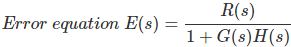Applying final value theorem, Steady state error = Lts→0 S E(s) Steady state error for different types and different inputs as follows: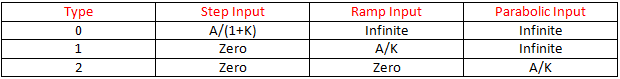07․ what is the Laplace transform of a function δ(t-2)?
2
0
2s
e-2s

L(δ(t)) = 1 Given function is δ(t-2), it represents the time delay by two units. It can be written as, L(δ(t-2)) = e-2s

08․ The impulse response of a linear system is e-t, (t > 0). The corresponding transfer function is
1/s(s+1)
1/(s+1)
1/s
s/(s+1)

Transfer function = L(impulse response) The transfer function in terms of Laplace transform of impulse response is known as weighting function. L(e-t) = 1/(s+1)

09․ A lead compensator used for a closed loop controller has the following transfer function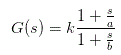For such a lead compensator
a < b.
b < a.
a > kb.
a < kb.

Zero at transfer function = - a, Pole at transfer function = - b. For a lead compensator, the zero is nearer to the origin as compared to the pole.

10․ The transfer functions of two compensators are given below. Which one is correct?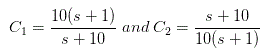which one is correct?
C1 is a lag compensator and C2 i
C1 is a lead compensator and C2 i
Both C1 and C2 are lead compensator.
Both C1 and C2 are lag compensator.

For a lead compensator, the zero is nearer to the origin and for lag compensator the pole is nearer to the origin. Here in C1 numerator (zero) s = - 1, denominator (pole) = - 10. So, s = - 1 is nearer to the origin. In C2 numerator (zero) s = - 10, denominator (pole) = - 1. So, s = - 10 is further from the origin. So, C1 is lead compensator and C2 is lag compensator.

<<<56789>>>